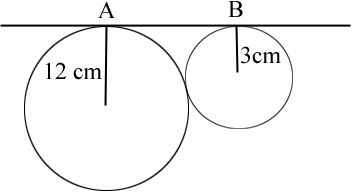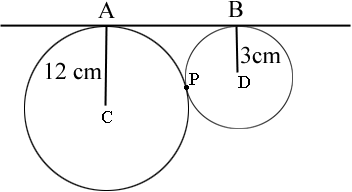SEARCH HOMEMath Central Quandaries & QueriesQuestion from Karan, a student: We are given 2 circles with radii 12cm and 3 cm. We have to find ABHere is the diagram Karen sentKaran,

I labeled some points on your diagram.P is the point where the circles are tangent.

Draw the line segments CP and PD. CPD is a straight line. Do you see why? If you draw one more line Pythagoras will give you the length of the line segment AB.

PennyMath Central is supported by the University of Regina and The Pacific Institute for the Mathematical Sciences.